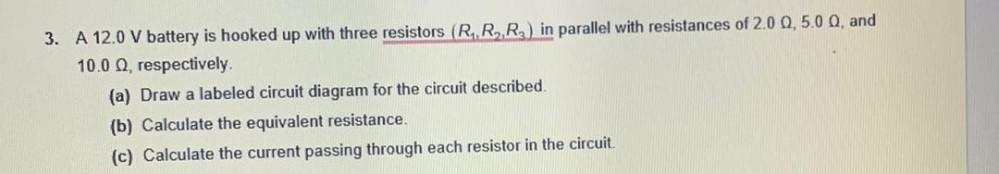Question:

# 3. A 12.0 V battery is hooked up with three resistors (R.,R,R2) in parallel with resistances of 20 0,50 0, and 10.0 , respective3. A 12.0 V battery is hooked up with three resistors (R.,R,R2) in parallel with resistances of 20 0,50 0, and 10.0 , respectively. (a) Draw a labeled circuit diagram for the circuit described (b) Calculate the equivalent resistance (c) Calculate the current passing through each resistor in the circuit